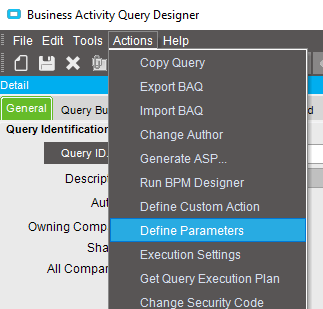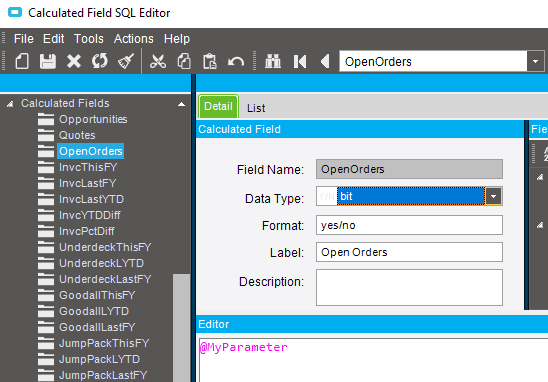# BAQ Criteria - Reference Parameter Only

Is it possible to have a criteria in a BAQ that is only evaluating a Parameter value, and doesn’t consider the value of any table/calculated field?

For example, if I have a boolean parameter, and I want to use it as a way to control my WHERE clause, so that it is only evaluated if that boolean parameter is true, can I do that in a BAQ?

In a SQL query I would write something like this:

``````DECLARE @AnyPart bit
DECLARE @PartNum nvarchar(50)

SELECT * FROM erp.Part
WHERE @AnyPart = 1 OR Part.PartNum = @PartNum
``````

I don’t know of a way to make the `WHERE @AnyPart = 1` part of the query in a BAQ.

There is a way to define parameters in a BAQ.Of course, but can you create a criteria that is only evaluating the parameter value, not the value of a table/field? In my example, I want to create a criteria that is equivalent to: `WHERE @MyBoolParameter = 1`. Note that there is no reference to a table/field or calculated field.

You can create a calculated field that is set to equal your parameter. Then you can add that to your subquery criteria.1 Like